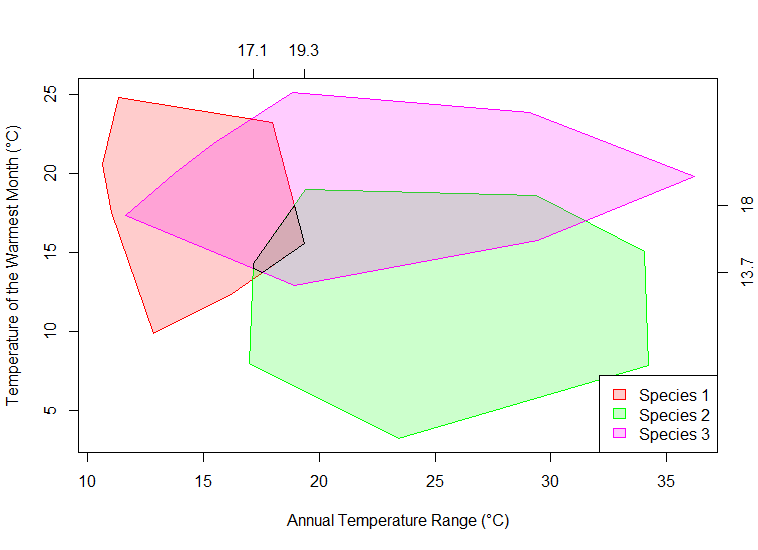# Illustrating the Mutual Climate Range (MCR) Method

I’m writing up some lecture material on transfer functions and environmental reconstruction, and needed to draw a diagram to illustrate the mutual climatic range method. This code could also be used to make simple MCR calculations and the like.An example of the output. Because the code produces random patterns, it doesn’t always produce overlapping ranges. It can simply be run a few times until the illustration looks good!

```# create 3 sets of normally distributed data x1 <- rnorm( 100, 15, 2 ) y1 <- rnorm( 100, 19, 2.5 ) # x2 <- rnorm( 100, 25, 4 ) y2 <- rnorm( 100, 12, 3 ) # x3 <- rnorm( 100, 25, 5 ) y3 <- rnorm( 100, 20, 2 ) # # compute the convex hull of those data h1 <- c( chull( x1, y1 ), chull( x1, y1 )[ 1 ] ) h2 <- c( chull( x2, y2 ), chull( x2, y2 )[ 1 ] ) h3 <- c( chull( x3, y3 ), chull( x3, y3 )[ 1 ] ) # # this library deals with polygon clipping require( polyclip ) # # compute the clipping area # this is a polyclip method inside another polyclip method # because polyclip is pairwise clip <- polyclip( polyclip( list( x = x1[ h1 ], y = y1[ h1 ] ), list( x = x2[ h2 ], y = y2[ h2 ] ) ), list( x = x3[ h3 ], y = y3[ h3 ] ) ) # # plot the polygons plot( 0, type = "n", xlim = c( range( c( x1, x2, x3 ) ) ), ylim = c( range( c( y1, y2, y3 ) ) ), xlab = "Annual Temperature Range (°C)", ylab = "Temperature of the Warmest Month (°C)" ) polygon( x1[ h1 ], y1[ h1 ], col = adjustcolor( "red", alpha.f = 0.2 ), border = "red" ) polygon( x2[ h2 ], y2[ h2 ], col = adjustcolor( "green", alpha.f = 0.2 ), border = "green" ) polygon( x3[ h3 ], y3[ h3 ], col = adjustcolor( "magenta", alpha.f = 0.2 ), border = "magenta" ) polygon( clip[[ 1 ]] ) # # add a legend legend( "bottomright", legend = c( "Species 1", "Species 2", "Species 3" ), fill = c( adjustcolor( "red", alpha.f = 0.2 ), adjustcolor( "green", alpha.f = 0.2 ), adjustcolor( "magenta", alpha.f = 0.2 ) ), border = c( "red", "green", "magenta" ) ) # # mark the mutual ranges on the opposing axes axis( 3, labels = round( c( min( clip[[ 1 ]]\$x ), max( clip[[ 1 ]]\$x ) ), 1 ), at = c( min( clip[[ 1 ]]\$x ), max( clip[[ 1 ]]\$x) ) ) axis( 4, labels = round( c( min( clip[[ 1 ]]\$y ), max( clip[[ 1 ]]\$y ) ), 1 ), at = c( min( clip[[ 1 ]]\$y ), max( clip[[ 1 ]]\$y) ) ) ```